General Aptitude - 1

# General Aptitude - 1

Test Description

## 20 Questions MCQ Test GATE Chemistry Mock Test Series | General Aptitude - 1

General Aptitude - 1 for Chemistry 2022 is part of GATE Chemistry Mock Test Series preparation. The General Aptitude - 1 questions and answers have been prepared according to the Chemistry exam syllabus.The General Aptitude - 1 MCQs are made for Chemistry 2022 Exam. Find important definitions, questions, notes, meanings, examples, exercises, MCQs and online tests for General Aptitude - 1 below.
Solutions of General Aptitude - 1 questions in English are available as part of our GATE Chemistry Mock Test Series for Chemistry & General Aptitude - 1 solutions in Hindi for GATE Chemistry Mock Test Series course. Download more important topics, notes, lectures and mock test series for Chemistry Exam by signing up for free. Attempt General Aptitude - 1 | 20 questions in 60 minutes | Mock test for Chemistry preparation | Free important questions MCQ to study GATE Chemistry Mock Test Series for Chemistry Exam | Download free PDF with solutions
 1 Crore+ students have signed up on EduRev. Have you?
*Answer can only contain numeric values
General Aptitude - 1 - Question 1

### The value of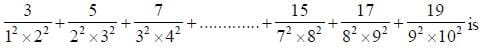_____________. (Round off two decimal places).

Detailed Solution for General Aptitude - 1 - Question 1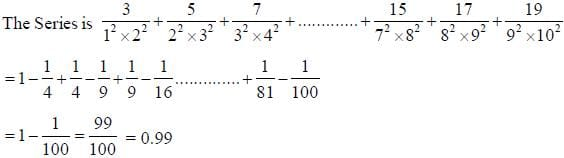General Aptitude - 1 - Question 2

### How many pieces of 85 cm can be cut from a rod of 42.5 metre

Detailed Solution for General Aptitude - 1 - Question 2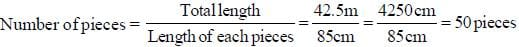General Aptitude - 1 - Question 3

### Find the missing number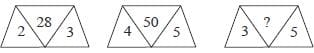Detailed Solution for General Aptitude - 1 - Question 3

1st 23 + 5 = 28
2nd 45 + 5 = 50
3rd 35 + 5 = 40

*Answer can only contain numeric values
General Aptitude - 1 - Question 4

If a = 0.1039, then the value of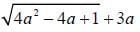is ___________ ( Round off to four decimal places)

Detailed Solution for General Aptitude - 1 - Question 4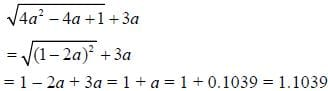General Aptitude - 1 - Question 5

Find the number of triangles in the figure.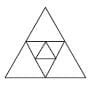Detailed Solution for General Aptitude - 1 - Question 5

As there are two triangles inside the large one. and each small triangle makes 4 triangles and the largest triangle makrs one. 4 x 2 + 1 = 9

General Aptitude - 1 - Question 6

a. b. c. d. e are five consecutive odd numbers, their average is

Detailed Solution for General Aptitude - 1 - Question 6

If 'a' is the odd no. then next consecutive odds are, a + 2, a + 4, a + 6, a + 8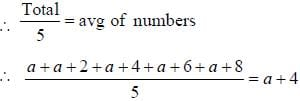[ If no observations are odd then the middle one is avg tor consecutive odd numbers.]

General Aptitude - 1 - Question 7

Introducing Asha to guests. Bhaskar said. "Her father is the only son of my father". How is Asha related to Bhaskar.

Detailed Solution for General Aptitude - 1 - Question 7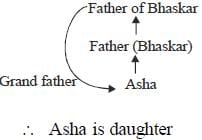General Aptitude - 1 - Question 8

The area of the largest triangle that can be inscribed in a semi circle of radius r is

Detailed Solution for General Aptitude - 1 - Question 8

As ΔBAC is on hill diameter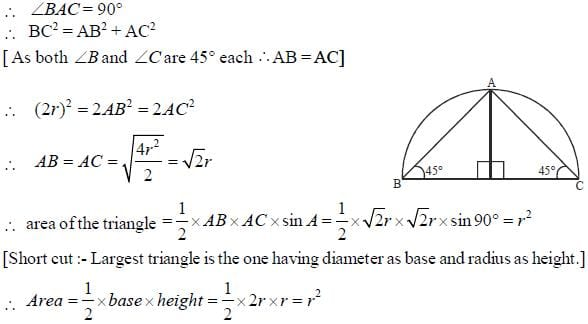General Aptitude - 1 - Question 9

A man had 16 dogs. All but 7 died. How many dogs was he left with

Detailed Solution for General Aptitude - 1 - Question 9

All but 7 died means except 7 dogs all others died i.e. 7 dogs are left

General Aptitude - 1 - Question 10

What angle is made by minute hand in 29 seconds?

Detailed Solution for General Aptitude - 1 - Question 10

In 3600 seconds it makes 360° (as if completes a circle)
∴ In 29 seconds it makes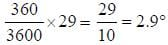General Aptitude - 1 - Question 11

A, B and C can do a piece of work in 20, 30 and 60 days respectively. In how many days A can do the work if he is assisted by B and C on every third day.

Detailed Solution for General Aptitude - 1 - Question 11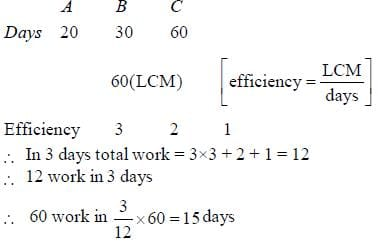General Aptitude - 1 - Question 12

Find the number of digits in 213

Detailed Solution for General Aptitude - 1 - Question 12

213 = ( 26)2 x 2 = (64)2 x 2 = (4096) x 2 = 8192 i.e. 4 digits

General Aptitude - 1 - Question 13

In the given figure AB is the diameter of the circle. Find the value o f  ∠A C D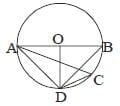Detailed Solution for General Aptitude - 1 - Question 13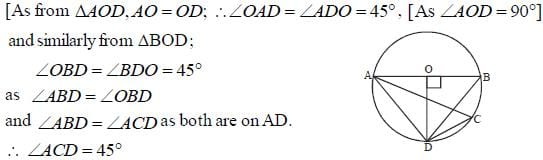General Aptitude - 1 - Question 14

The minute hand of a clock is in North -East at 8 : 45. Then in which direction the hour hand will be at 1 : 30.

Detailed Solution for General Aptitude - 1 - Question 14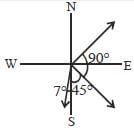So. 1 : 30 and 8 : 45 difference = 4 h o u r : 45 mins =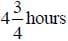and by that time clock's hour hand will move forward . in 12 horns, hour hand makes 360°.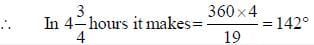General Aptitude - 1 - Question 15

Bombay express left delhi for bomb ay at 14: 30 hours, travelling at a speed of 60 km hr. and Rajdhani express left delhi for bombay at 16:30 hours on the same day at a speed of 80 km hr. How for from delhi the two trains will meet?

Detailed Solution for General Aptitude - 1 - Question 15

In two hours first train goes 120 km. (as speed = 60 km/hr)
Now relative speed = (80 - 60) km hour = 20 km hour.
Now 20 km is covered in 1 hour.
∴ 120 km is convered in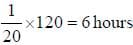Which is the time for the 2nd train which travels at 80 km hour
∴ Total distance covered = 80 x 6 = 480 km.

General Aptitude - 1 - Question 16

A dishonest shop keeper sells sugar at cost price but cheats 10% on weight. What is his percentage profit loss

Detailed Solution for General Aptitude - 1 - Question 16

Let cost price of 100 g is 100 Rs.
∴ The cost price of 90 g is 90 Rs.
∴ he profits 10 Rs in each 90 Rs.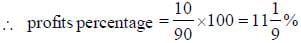General Aptitude - 1 - Question 17

Statement
(i) All A are B
(ii) All A are C (Hi) Some C are D
Based on these statements find out which at the following conclusions are true.
(1) All c can be B
(2) All A are B as well as C.

Detailed Solution for General Aptitude - 1 - Question 17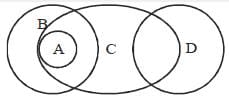This is the general diagram. But C can also be inside B. which is a possibility,
∴ both follows (c)

General Aptitude - 1 - Question 18

Find out in the given sentese if there is any error and mark the part having error as your answer, and if No error is there then mark 'd' as your answer.
(i) All the students of the academy will
(ii) Give a test
(iii) O f general aptitude in the afternoon
(iv) No error

Detailed Solution for General Aptitude - 1 - Question 18

’give a test' is wrong conect is ’take a test'.

*Answer can only contain numeric values
General Aptitude - 1 - Question 19

The reina inder o f the series l!+ 2 !+ 3 !+ 4 !+ + 100! when divided by 10 is____________.

Detailed Solution for General Aptitude - 1 - Question 19

l!+2!+3!+4! = 1 + 2 + 6+24 = 33
and each te rm > 5!. should have a 2 and 5 is the no (i.e. 5! = lx 2 x 3 x 4 x 5 )
Which is divisible by 10.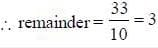General Aptitude - 1 - Question 20

There are 8 identical bags of wheat except 1 bag all others have same weight but one bag is heavier what is the minimum number of weights requited to identify the bag with different weight by conventional balance.

Detailed Solution for General Aptitude - 1 - Question 20

Total 8 bags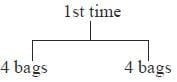take which one is heavier, next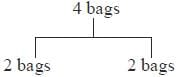again take heavier one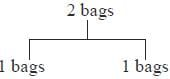## GATE Chemistry Mock Test Series

1 docs|34 tests
 Use Code STAYHOME200 and get INR 200 additional OFF Use Coupon Code
Information about General Aptitude - 1 Page
In this test you can find the Exam questions for General Aptitude - 1 solved & explained in the simplest way possible. Besides giving Questions and answers for General Aptitude - 1, EduRev gives you an ample number of Online tests for practice

1 docs|34 tests